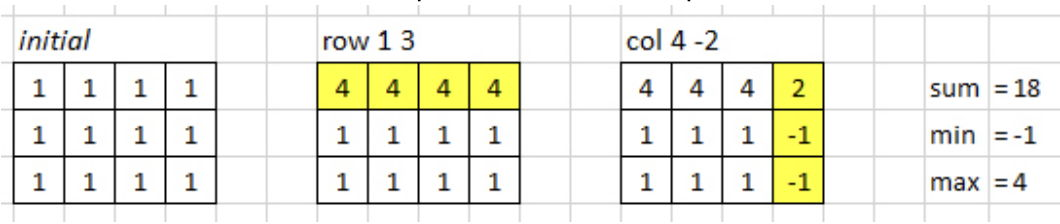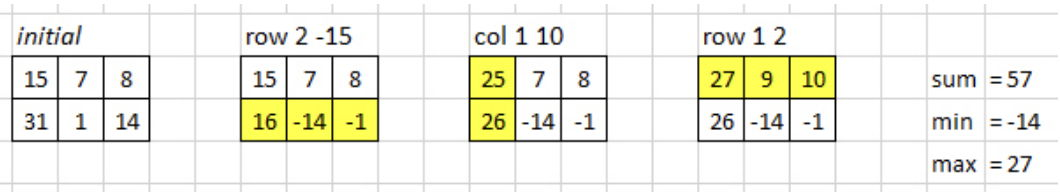시간 제한 메모리 제한 제출 정답 맞은 사람 정답 비율
1 초 512 MB 17 17 17 100.000%

## 문제

Matrix is a mathematical object which arranges data into a rectangular array of N rows and M columns. The rows are indexed from 1 to N, while the columns are indexed from 1 to M. Matrix is very powerful and extremely useful in many applications. In this problem, we are going to focus on two simple operations in matrix: row addition and column addition.

You are given a matrix of integers of N rows and M columns, and Q queries of the following format:

• row k val: add each element on the k-th row by val,
• col k val: add each element on the k-th column by val.

Your task is to output the following three numbers after all queries have been performed:

• sum: the sum of all elements in the matrix,
• min: the value of the smallest element in the matrix,
• max: the value of the largest element in the matrix.

See the sample input for clarity

## 입력

The first line contains two integers: N M (1 ≤ N, M ≤ 50) denoting the size of the matrix (number of rows and columns, respectively). The next N lines, each contains M integers: Ai,j (-100 ≤ Ai,j ≤ 100) denoting the matrix element at the i-th row and j-th column for 1 ≤ iN and 1 ≤ jM, respectively. The next line contains an integer: Q (0 ≤ Q ≤ 100) denoting the number of queries. The next Q lines, each contains a query in one of the following format:

• row k val (1 ≤ kN; -100 ≤ val ≤ 100)
• col k val (1 ≤ kM; -100 ≤ val ≤ 100)

## 출력

The output contains three integers (each separated by a single space) in a single line: sum min max, as described in the problem statement.

## 예제 입력 1

3 4
1 1 1 1
1 1 1 1
1 1 1 1
2
row 1 3
col 4 -2


## 예제 출력 1

18 -1 4


These are the matrices after each queries for the first sample.## 예제 입력 2

4 3
10 10 10
10 10 10
10 10 10
10 10 10
5
row 2 -5
col 3 6
col 1 -10
row 4 7
col 1 3


## 예제 출력 2

122 -2 23


## 예제 입력 3

2 3
15 7 8
31 1 14
3
row 2 -15
col 1 10
row 1 2


## 예제 출력 3

57 -14 27


These are the matrices after each queries for the third sample.